## Chemical Equilibrium Questions and Answers Part-10

1. Consider the reaction equilibrium
$2 SO_{2}\left(g\right)+O_{2}\left(g\right)\rightleftharpoons 2 SO_{3}\left(g\right); \triangle H^{\circ}=-198 kJ$
On the basis of Le Chatelier’s principle, the condition favourable for the forward reaction is
a) increasing temperature as well as pressure
b) lowering the temperature and increasing the pressure
c) any value of temperature and pressure
d) lowering of temperature as well as pressure

Explanation: Due to exothermicity of reaction low or optimum temperature will be required. Since 3 moles are changing to 2 moles.
High pressure will be required

2.What is the equilibrium expression for the reaction $P_{4\left(s\right)}+5O_{2\left(g\right)}\rightleftharpoons P_{4}O_{10\left(s\right)}$
a) $K_{C}=\left[O_{2}\right]^{5}$
b) $K_{C}=\left[P_{4}O_{10}\right] / 5\left[P_{4}\right]\left[O_{2}\right]$
c) $K_{C}=\left[P_{4}O_{10}\right]/\left[P_{4}\right]\left[O_{2}\right]^{5}$
d) $K_{C}=1/\left[O_{2}\right]^{5}$

Explanation: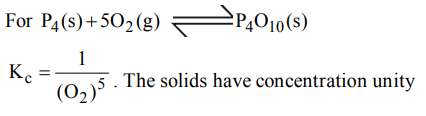3. For the reaction, $CO_{\left(g\right)}+Cl_{2\left(g\right)}\rightleftharpoons COCl_{2\left(g\right)}$      the $K_{P}\diagup K_{C}$   is equal to
a) $\sqrt{RT}$
b) RT
c) $1\diagup RT$
d) 1.0

Explanation: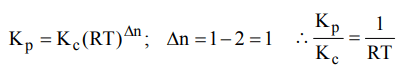4. In a cell that utilises the reaction $Zn_{\left(s\right)}+2H^{+}_{\left(aq\right)} \rightarrow Zn^{2+}_{\left(aq\right)}+H_{2\left(g\right)}$      addition of $H_{2}SO_{4}$  to cathode compartment, will
a) increase the E and shift equilibrium to the right
b) lower the E and shift equilibrium to the right
c) lower the E and shift equlibrium to the left
d) increase the E and shift equilibrium to the left

Explanation: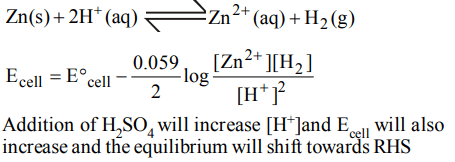5. The exothermic formation of $ClF_{3}$  is represented by the equation :
$Cl_{2\left(g\right)}+3F_{2\left(g\right)}\rightleftharpoons 2ClF_{3\left(g\right)}; \triangle H = – 329 kJ$
Which of the following will increase the quantity of $ClF_{3}$   in an equilibrium mixture of $Cl_{2}, F_{2} and ClF_{3}$    ?
a) Adding $F_{2}$
b) Increasing the volume of the container
c) Removing $Cl_{2}$
d) Increasing the temperature

Explanation: The reaction given is an exothermic reaction thus according to Le chatalier’s principle lowering of temperature, addition of F2 and Cl2 favour the forward direction and hence the production of ClF3 .

6. For the reaction
$2NO_{2\left(g\right)}\rightleftharpoons 2NO_{\left(g\right)} +O_{2\left(g\right)}$
$\left(K_{C}=1.8\times10^{-6} at 184°C\right)$      (R = 0.0831 kJ/ (mol. K))
When $K_{P} and K_{C}$   are compared at 184°C, it is found that
a) Whether$K_{P}$ is greater than, less than or equal to $K_{C}$ depends upon the total gas pressure
b) $K_{P} = K_{C}$
c) $K_{P}$  is less than  $K_{C}$
d) $K_{P}$  is greater than  $K_{C}$

Explanation: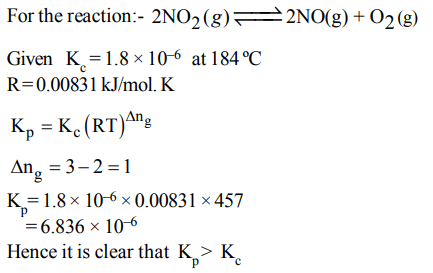7. An amount of solid $NH_{4} HS$   is placed in a flask already containing ammonia gas at a certain temperature and 0.50 atm pressure. Ammonium hydrogen sulphide decomposes to yield $NH_{3} and H_{2}S$    gases in the flask. When the decomposition reaction reaches equilibrium, the total pressure in the flask rises to 0.84 atm? The equilibrium constant for $NH_{4} HS$   decomposition at this temperature is
a) 0.11
b) 0.17
c) 0.18
d) 0.30

Explanation: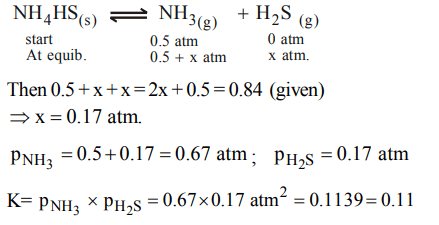8. The equilibrium constant for the reaction
$SO_{3} \left(g\right)\rightleftharpoons SO_{2} \left(g\right)+\frac{1}{2}O_{2} \left(g\right)$
is $K_{C} =4.9 ×10^{-2}$   . The value of $K_{C}$  for the reaction
$2SO_{2} \left(g\right)+O_{2} \left(g\right)\rightleftharpoons 2SO_{3} \left(g\right)$
will be
a) $9.8 × 10^{-2}$
b) $4.9 × 10^{-2}$
c) 416
d) $2.40 × 10^{-3}$

Explanation: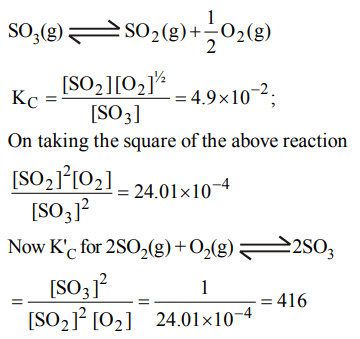9. The equilibrium constants $K_{P1} and K_{P2}$  for the reactions $X\rightleftharpoons 2Y$  and  $Z\rightleftharpoons P+Q$  respectively are in the ratio of 1 : 9. If the degree of dissociation of X and Z be equal then the ratio of total pressures at these equilibria is
a) 1 : 1
b) 1: 36
c) 1 : 3
d) 1 : 9

Explanation: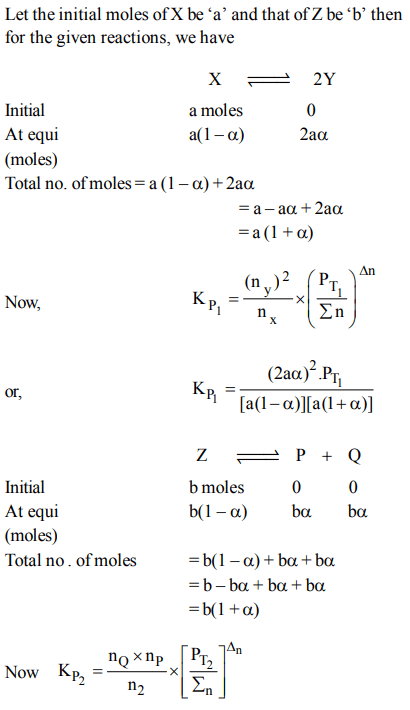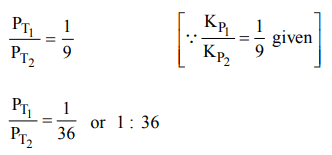10. For the following three reactions a, b and c, equilibrium constants are given:
(i)$CO\left(g\right) + H_{2}O\left(g\right)\rightleftharpoons CO_{2}\left(g\right) + H_{2}\left(g\right);K_{1}$
(ii)$CH_{4}\left(g\right) + H_{2}O\left(g\right)\rightleftharpoons CO\left(g\right) + 3H_{2}\left(g\right);K_{2}$
(iii)$CH_{4}\left(g\right) + 2 H_{2}O\left(g\right)\rightleftharpoons CO_2\left(g\right) + 4H_{2}\left(g\right);K_{3}$
a) $K_{1}\sqrt{K_{2}}=K_{3}$
b) $K_{2}K_{3}=K_{1}$
c)$K_{3}=K_{1}K_{2}$
d) $K_{3}K_2^3=K_1^2$